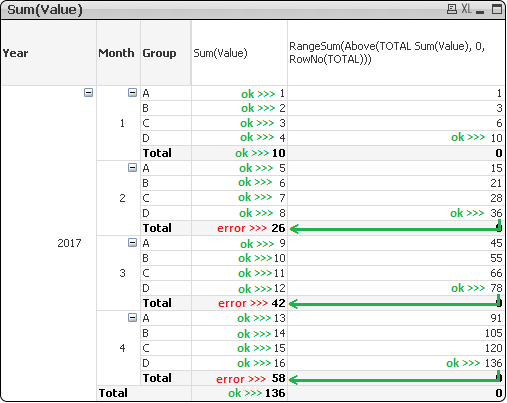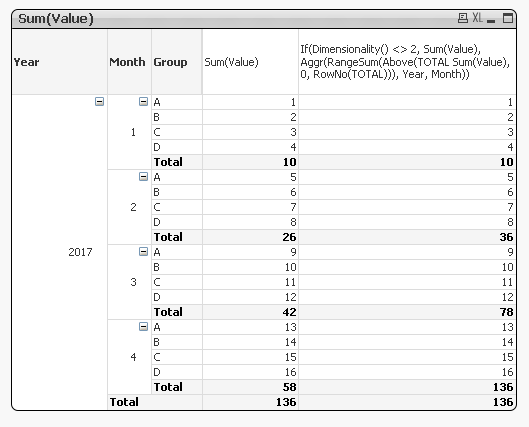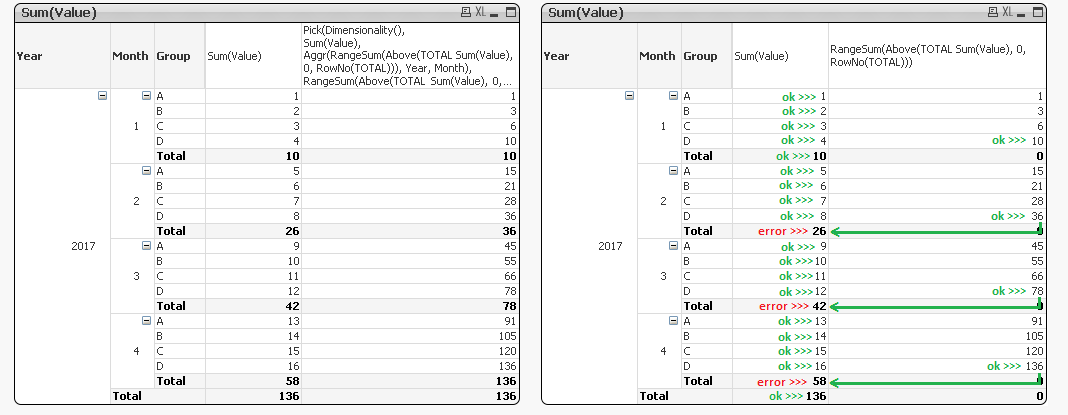# QlikView App Development

Discussion Board for collaboration related to QlikView App Development.

Highlighted
Contributor III

## Accumulate Partial Sum on Pivot Table

Hi all,

I have a pivot table and I try to obtain a progressive value on Total rows as explained in the image.

I attached a *.qvwThose are a simple data values.

```T:
Year, Month, Group, Value
2017, 1, A, 1
2017, 1, B, 2
2017, 1, C, 3
2017, 1, D, 4
2017, 2, A, 5
2017, 2, B, 6
2017, 2, C, 7
2017, 2, D, 8
2017, 3, A, 9
2017, 3, B, 10
2017, 3, C, 11
2017, 3, D, 12
2017, 4, A, 13
2017, 4, B, 14
2017, 4, C, 15
2017, 4, D, 16
];
```

I hope someone can help me.

Best Regards

Luca Jonathan Panetta

1 Solution

Accepted SolutionsMVP

## Re: Accumulate Partial Sum on Pivot Table

This:If(Dimensionality() <> 2, Sum(Value),

Aggr(RangeSum(Above(TOTAL Sum(Value), 0, RowNo(TOTAL))), Year, Month))

6 RepliesMVP

## Re: Accumulate Partial Sum on Pivot Table

Try this:

Pick(Dimensionality(),

Sum(Value),

Aggr(RangeSum(Above(TOTAL Sum(Value), 0, RowNo(TOTAL))), Year, Month),

RangeSum(Above(TOTAL Sum(Value), 0, RowNo(TOTAL))))Contributor III

## Re: Accumulate Partial Sum on Pivot Table

Hi Sunny,

I want to have the value in column 1 for A, B, C, D and the cumulative value for Total.

The correct values will be

20171A1
B2
C3
D4

2A5
B6
C7
D8

3A9
B10
C11
D12

4A13
B14
C15
D16

### 136

Regards

Luca Jonathan PanettaMVP

## Re: Accumulate Partial Sum on Pivot Table

This:If(Dimensionality() <> 2, Sum(Value),

Aggr(RangeSum(Above(TOTAL Sum(Value), 0, RowNo(TOTAL))), Year, Month))

Contributor III

## Re: Accumulate Partial Sum on Pivot Table

I found solution.

Pick(Dimensionality(),

Sum(Value),

Aggr(RangeSum(Above(TOTAL Sum(Value), 0, RowNo(TOTAL))), Year, Month),

Sum(Value)

)

Thanks Sunny for your help. I have change only the 3th expression from RangeSum(Above(TOTAL Sum(Value), 0, RowNo(TOTAL))) to Sum(Value)

Best regards.

Luca Jonathan Panetta

Contributor III

## Re: Accumulate Partial Sum on Pivot Table

Thanks Sunny.

But I like more Pick function than not IF.

Best regards

Luca Jonathan PanettaMVP

## Re: Accumulate Partial Sum on Pivot Table

If you like Pick more, you can always mark your own response as correct. You can mark my responses as Helpful if you want (you don't have to)

Qlik Community Tip: Marking Replies as Correct or Helpful

Best,

Sunny